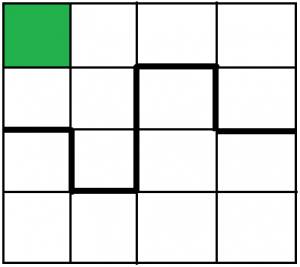GFG App
Open AppBrowser
Continue

# Check if any square (with one colored cell) can be divided into two equal parts

Given a square of size n. There are n2 small squares inside the square n of size 1 unit each, in which any one of the squares is colored. Our task is to cut the square n into two equal parts. The cutting line should not have any common points with the colored cell and the resulting two parts should be equal up to rotation. Print “YES” if it’s possible to cut the square with such conditions and “NO” otherwise.
Note: The value of n should always be an even positive number.

Examples:

```Input : n = 4, x = 1, y = 1
Output : YES
// n = 4 and 1 1 is the coordinate of the colored square

Input :  n = 2, x = 1, y = 1
Output : NO ```In the first example, the painted square has a coordinate 1 x 1. So we have to cut the bigger square into two parts so that there should not be any common point with the colored cell. The bold line shown in the above picture cuts the square into two equal parts.
Below is the step by step algorithm to solve this problem:
1 . Initialize the size of the square and the position of the painted square.
2 . Dividing a square into two equal parts will only be possible if the cutting line passes through the center of our bigger square.
3 . Thus, if the painted square is linked anyway to the center of the bigger square, then it is not possible to cut the bigger square into two equal parts.
4 . So to check, divide the size of the bigger square into half and check if any dimension of a painted square is linked to it.

Below is the implementation of the above approach:

## C++

 `// C++ program to illustrate` `// the above problem`   `#include ` `using` `namespace` `std;`   `// function to check if it's possible to` `// divide the square in two equal parts` `void` `halfsquare(``int` `n, ``int` `x, ``int` `y)` `{` `    ``int` `half = n / 2;`   `    ``// if the painted square is linked anyway` `    ``// to the center of the square` `    ``// then it's not possible` `    ``if` `((half == x || half == x - 1) && ` `        ``(half == y || half == y - 1))` `        ``cout << ``"NO"` `<< endl;`   `    ``// else yes it's possible` `    ``else` `        ``cout << ``"YES"` `<< endl;` `}`   `// Driver code` `int` `main()` `{` `    ``// initialize the size of the square` `    ``int` `n = 100;`   `    ``// initialize the dimension of the painted square` `    ``int` `x = 51, y = 100;`   `    ``halfsquare(n, x, y);` `    ``return` `0;` `}`

## Java

 `// Java program to illustrate` `// the above problem`   `import` `java.io.*;`   `class` `GFG {` ` `  `// function to check if it's possible to` `// divide the square in two equal parts` `static` `void` `halfsquare(``int` `n, ``int` `x, ``int` `y)` `{` `    ``int` `half = n / ``2``;`   `    ``// if the painted square is linked anyway` `    ``// to the center of the square` `    ``// then it's not possible` `    ``if` `((half == x || half == x - ``1``) && ` `        ``(half == y || half == y - ``1``))` `        ``System.out.println( ``"NO"``);`   `    ``// else yes it's possible` `    ``else` `        ``System.out.println( ``"YES"``);` `}`   `// Driver code`   `    ``public` `static` `void` `main (String[] args) {` `            ``// initialize the size of the square` `    ``int` `n = ``100``;`   `    ``// initialize the dimension of the painted square` `    ``int` `x = ``51``, y = ``100``;`   `    ``halfsquare(n, x, y);` `    ``}` `}` `// This code is contributed ` `// by inder_verma..`

## Python 3

 `# Python 3 program to illustrate ` `# the above problem `   `# function to check if it's possible to ` `# divide the square in two equal parts ` `def` `halfsquare(n, x, y) :` `    ``half ``=` `n ``/``/` `2`   `    ``# if the painted square is ` `    ``# linked anyway to the center ` `    ``# of the square then it's ` `    ``# not possible ` `    ``if` `((half ``=``=` `x ``or` `half ``=``=` `x ``-` `1``) ``and` `        ``(half ``=``=` `y ``or` `half ``=``=` `y ``-` `1``)) :` `        ``print``(``"NO"``)`   `    ``# else yes it's possible ` `    ``else` `:` `        ``print``(``"YES"``)` `        `  `# Driver code     ` `if` `__name__ ``=``=` `"__main__"` `:`   `    ``# initialize the size of the square ` `    ``n ``=` `100`   `    ``# initialize the dimension ` `    ``# of the painted square ` `    ``x, y ``=` `51``, ``100`   `    ``halfsquare(n, x, y)`   `# This code is contributed by ANKITRAI1`

## C#

 `// C# program to illustrate` `// the above problem` `using` `System;`   `class` `GFG {`   `// function to check if it's possible to` `// divide the square in two equal parts` `static` `void` `halfsquare(``int` `n, ``int` `x, ``int` `y)` `{` `    ``int` `half = n / 2;`   `    ``// if the painted square is linked anyway` `    ``// to the center of the square` `    ``// then it's not possible` `    ``if` `((half == x || half == x - 1) && ` `        ``(half == y || half == y - 1))` `        ``Console.WriteLine( ``"NO"``);`   `    ``// else yes it's possible` `    ``else` `        ``Console.WriteLine( ``"YES"``);` `}`   `// Driver code`   `    ``public` `static` `void` `Main () {` `            ``// initialize the size of the square` `    ``int` `n = 100;`   `    ``// initialize the dimension of the painted square` `    ``int` `x = 51, y = 100;`   `    ``halfsquare(n, x, y);` `    ``}` `}` `// This code is contributed ` `// by  anuj_67..`

## PHP

 ``

## Javascript

 ``

Output:

`YES`

Time complexity: O(1) because it is performing constant operations

Auxiliary Space: O(1)

My Personal Notes arrow_drop_up